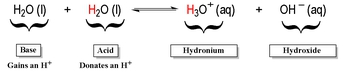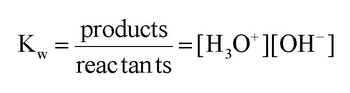## GOB Chemistry

Learn the toughest concepts covered in your GOB - General, Organic, and Biological Chemistry class with step-by-step video tutorials and practice problems.

Acids and Bases

# Auto-Ionization

In a Self-Ionization reaction two water molecules react with one another, where one acts an acid and the other as a base.

1
concept

## Auto-Ionization Concept 13m
Play a video:
Hey, guys, In this new video, we're gonna take a look at the auto ionization of water. So what we're gonna say here is in an acquis solution. So the solvent is water. Water molecules can react with each other on. We're gonna say this is called the process off auto ionization or self ionization. Now, in this process, we're going to create H 30 plus and O H minus. Now just realize, how does this happen for this to occur? One of the water molecules has to act as an acid, and the other one has to act as a base. So let's say that this one here acts as the acid, and this year is the base. Remember, Bronston Laurie definitions. The acid acts as an H plus donor. The base acts as an H plus except er, so this base right here is going to accept an H plus, and as a result, it's gonna become H 30 plus. Then we're gonna say that the other water molecule, the one that's acting as an acid because it's giving away an H plus it becomes O. H minus and just also realize here that water molecules exists in liquid form. But then once they become these ions the hydro Nia, my on and the hydroxide ion they're gonna become a quiz. So they're no longer liquid water. And because of that, they're no longer a liquid. There gonna be a quiz? Remember, A quiz just means that the parts of the water are attracted to these particular ions. We can simplify auto ionization or self organization by just saying that water separates into H plus plus O H minus. Remember, we've said this before that h plus is the same thing as H 30 plus. Okay, so both are hydro Nia, my on They both mean the same exact thing. Now we're gonna say here that the equilibrium equation for water is called the ion product and I on product, it's simply said to bkw So when we're talking about the k w for water, we're talking about the ion product of water and we're gonna say that K w equals your hydro knee my on multiply times, your hydroxide ion, and we're gonna say here that at 25 degrees Celsius, K W is 1.0 times 10 to the negative 14. But remember, Just like all case, it's going to be temperature dependent. If you change the temperature, you're gonna change the value of the K W. So we're gonna say increasing the temperature will increase our kw. Now, here I give us a bunch of different values for K W, found at various temperatures and notice as a temperature. Gradually increases are K W will get bigger and bigger now. Just realize on your exam when you need to use K W. If they don't give you a temperature, just assume that it's 25 degrees Celsius. So then K W would be 1.0 times 10 to the negative 14. Now, knowing this, let's take a look at this question here.The ionization product of Kw is used in this self-ionization equation and ignoring solids and liquids gives the equilibrium equation as:2
example

## Auto-Ionization Example 12m
Play a video: